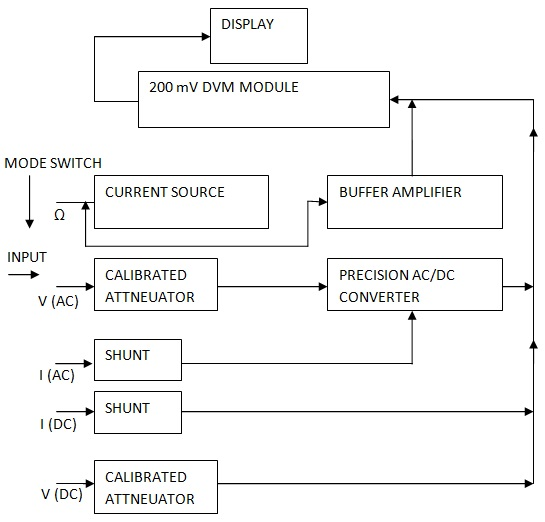0

Digital Multimeter Block Diagram Explanation

• A digital multimeter is one that is capable of measuring voltage, current, of alternating current circuits as well as direct current circuits. In addition it must have a provision for measurement of resistance also. Then only it becomes the digital AVO meter.

As far as voltage measurement is concerned we have seen above the blocks required. For resistance measurement and current measurement we have to know the procedure. Both are indirect methods of measurement. We will be measuring only the voltage. However the reading can be made in amperes or ohms, when switched on to these modes.

(a) Block Diagram of Digital Multimeter :

The block diagram of digital multimeter is shown below. The 200 mV module is shown as one block. The rest of the blocks are interconnected to the 200 mV module as shown in Figure.Block Diagram of a Digital Multimeter

(b) Explanation of the Block Diagram :

The block diagram shows that the input terminal is connected to a mode selector switch. The other input terminal is a common terminal, generally referred as ground or negative terminal. The mode selector switch has five positions. Each position of the switch is marked with its function. In the first position of the switch the input goes to ohms select. ln this mode the input to the multimeter is an unknown resistance. As the unknown resistance has to form a part of the potential divider with the internal constant current source and range multiplier resistor, it is shown to be connected to the buffer amplifier. At the same time the block constant current generator is linked with the input terminal. Therefore the buffer amplifier will amplify only the voltage that is developed across the unknown resistance. The unknown resistance and the internal range resistor form a potential divider using the current from the constant current source. Hence the resistance to voltage conversion is complete and the D.V.M. reads the resistance.

The second position of the range switch is marked as Volts (A.C.). The input signal as an unknown alternating voltage will go to the calibrated attenuator. The output of the attenuator will go to the precision AC to DC converter. The output of the AC/DC convener will go, to the DVM module. Selecting the proper range we can measure alternating voltage.

The third position of the switch is marked as current (A.C.). Hence the unknown current is to be measured. The internal shunt carries unknown current and the voltage developed across it will be converted in to steady voltage D.C.( voltage), by the AC/DC converter. As the converter’s output is given to the D.V.M, module the current is displayed. Different shunts will be selected by a range selector used with the shunt circuit.

In the fourth position the direct current can be measured. The unknown current flows through the selected shunt (range selector is used). The voltage developed across this shunt goes to D.V.M. module. So in the fourth position of the mode selector we will be able to measure direct current. The fifth position is marked as volts D.C. The input is the unknown voltage. This voltage passes through a calibrated attenuator.

The range selection is done. The output of the attenuator goes to the D. V M. Thus the steady voltage or D.C. voltage can be measured. The other blocks that are not shown in the above simple block diagram are auto range circuit, auto polarity circuit, power supply, component testing circuit.

It is to be remembered that the mode selection and range selection switches, and the arrangement differ from make to make as per design specifications of the manufacturer.